# Metastock to MT4744

MetaStock code for creating the PFE indicator:

Polarized Fractal Efficiency

Mov(If(C,>,Ref(C,-9),Sqr(Pwr(Roc(C,9,\$),2) + Pwr(10,2)) /

Sum(Sqr(Pwr(Roc(C,1,\$),2)+1),9),-

Sqr(Pwr(Roc(C,9,\$),2) + Pwr(10,2)) /

Sum(Sqr(Pwr(Roc(C,1,\$),2)+1),9))*100,5,E)

CAN ANY ONE TRY TO CODIT FOR META TRADER 4 THANKS46

Files:367

A code of the MT3

I coded this from the MT3 code above. It is different than the one that you've posted - that one came from a russian forum as I understand it and isn't based on the MT3 code.

I don't have MT3 to test this for validity - do you? If so, I'd love to know if this does what you are looking for.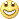Cheers,

CS

Files:25

Metastock to MT4

The code below is metastock format, Can someone help to convert to MT4 ? with option mov preriods change.

It's trend following oscillator, it's can use with break out strategies like price chanel or price chanal with ATR adjusted to make trading system.(see attached picture for a sample system)

Bull Power/Bear Power Histogram

r1:=If(Ref(C,-1)<O,Max(O-Ref(C,-1),H-L),H-L);

r2:=If(Ref(C,-1)>O,Max(Ref(C,-1)-O,H-L),H-L);

bull:=If(C=O,

If(H-C=C-L,

If(Ref(C,-1)>O,

Max(H-O,C-L),

r1),

If(H-C>C-L,

If(Ref(C,-1)<O,

Max(H-Ref(C,-1),C-L),

H-O),

r1)),

If(C<O,

If(Ref(C,-1)<O,

Max(H-Ref(C,-1),C-L),

Max(H-O,C-L)),

r1));

bear:=If(C=O,

If(H-C=C-L,

If(Ref(C,-1)<O,

Max(O-L,H-C),

r2),

If(H-C>C-L,

r2,

If(Ref(C,-1)>O,

Max(Ref(C,-1)-L,H-C),

O-L))),

If(C<O,

r2,

If(Ref(C,-1)>O,

Max(Ref(C,-1)-L,H-C),

Max(O-L,H-C))));

BB:=Mov(bull-bear,30,S); { need option mov preriods change}

slowBB:= mov(BB,89,s); { need option mov preriods change}

fastBB:= mov(BB,13,s); { need option mov preriods change}

Thank you

Files: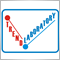7016

Hi,

It's so-called Bull And Bear Balance Indicator by Vadim Gimelfarb.

And looks very similar to MACD.

Igor

Files: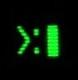422

Thanks Igorad104

bugkeab:

hi

were this indicators Attached Thumbnails plz25

Thank you very much Igorad.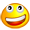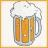158

Hi, I figured I would use this indicator too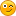I attempted to convert it to MT4 from VTrader... here's the original code:

_pfe:= Mov(If(pr>Ref(pr,-length),sqrt(Power(Roc(pr,length,Points),2) + Power(10,2)) /

Sum(Sqrt(Power(Roc(pr,1,Points),2)+1),length),-

Sqrt(Power(Roc(pr,length,Points),2) + Power(10,2)) /

Sum(Sqrt(Power(Roc(pr,1,Points),2)+1),length))*100,smooth,E);

And the MT4 version is in the attachment. However, it doesn't look like the original one (check the picture), the original one is smoother. Plus, I had to put a fixed period because when it was a variable, it always returned zero.

I'm a bad programmer, so I ask anyone willing to help to check the MT4 code and correct the errors, generally it should be somewhat right.

And, as you can see from the picture, it's worth it to have this indicator in MT4, very useful, so do it for your own goodThanks!

Files:
pfe.gif 14 kb253

correct setup to Metastock (METASTOCK, NOT METATRADER) to backtest

there is not forum for this, but i know that many respected traders like Igor uses Metastock saying that is far more reliable than Metatrader.

so what is the correct setup to do that?

if is it correct that Metastock is really reliable, so i think that this topic would be very useful.

sorry for my very poor English.15

Help Needed to convert a metastock code to Mq4

I'm seeking for a help to convert the following 3 lines of MetaStock Code to MQ4 script

BB:=Mov( (H-L),15,S);

SS:=StochMomentum(5,3,3)< LLV(StochMomentum(5,3,3), 15);

If(Ref(SS, -1) AND C-O>BB,1, 0)